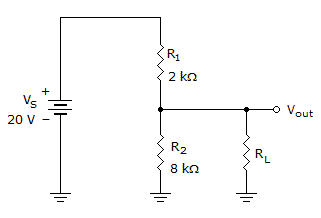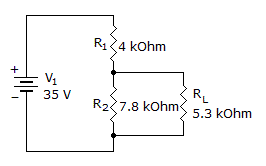# Electronics - Series-Parallel Circuits

### Exercise :: Series-Parallel Circuits - General Questions

16.

When placed into a circuit, how are electronic components usually connected?

 A. positive terminal to positive terminal B. in parallel C. as a combination of series and parallel D. in series

Explanation:

No answer description available for this question. Let us discuss.

17.If the load in the given circuit is 12 k, what is the loaded output voltage?

 A. 5.88 V B. 14.12 V C. 16 V D. 17.14 V

Explanation:

No answer description available for this question. Let us discuss.

18.

If a Wheatstone bridge is balanced, a voltmeter in the center of the bridge will read:

 A. the same as the source voltage B. half the source voltage C. zero volts D. twice the source voltage

Explanation:

No answer description available for this question. Let us discuss.

19.

What is the voltage to the load?A. 11.4 V B. 12.4 V C. 13.4 V D. 15.4 V

Explanation:

No answer description available for this question. Let us discuss.

20.

The first goal to accomplish in analyzing a complex series-parallel circuit is to

 A. equate all parallel components B. equate all series components C. solve for all the voltage drops D. solve for the total current and resistance